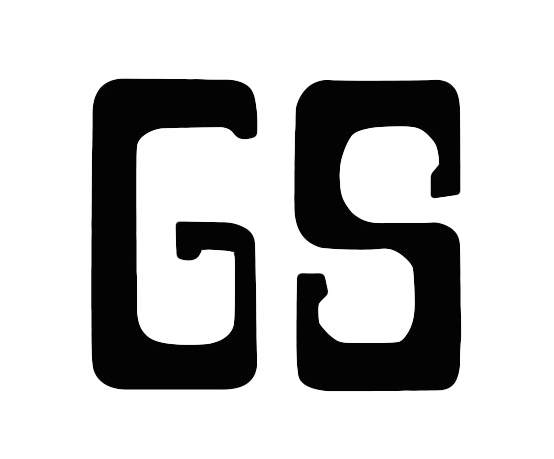by Corey

• articles

### Tags

• Bioinformatics
• R

The medical statistical data can be divided into numerical and categorical types. When there is a need to find out the association between numerical variables (age, weight), the t-test is fine. When we need to determine the association between categorical variables, like whether the gender is correlated with smoking status (example data in the contingency table below), we could use Chi-Squared test if the data is fit in with the prerequisite. Otherwise, we should use Fisher’s Exact Test.

Gender Smoker Non-Smoker
Male 20 3
Female 8 22

The test statistic for the Chi-Square Test of independence is denoted as $$\chi^{2}$$, and is computed as:

$\chi^{2} = \sum_{i=1}^{R}{\sum_{j=1}^{C}{\frac{(o_{ij} - e_{ij})^{2}}{e_{ij}}}}$

where $$o_{ij}$$ is the observed cell count in the $$i^{th}$$ row and $$j^{th}$$ column of the table $$e_{ij}$$ is the expected cell count in the $$i^{th}$$ row and $$j^{th}$$ column of the table, computed as

$e_{ij} = \frac{(\text{Row i Total})\times(\text{Col j Total})}{\text{Grand Total}}$

The condition is based on the N and $$e_{ij}$$:

1. N ≥ 40 and $$e_{ij}$$ ≥ 5. Use the Chi-Squared Test.
2. N ≥ 40 and 1 ≤ $$e_{ij}$$ ≤ 5. Use the Chi-Squared Test with correction (chisq.test with parameter correct=TRUE).
3. N < 40 or $$e_{ij}$$ < 1 or p-value close to 0.05. Can not use the Chi-Squared Test, should use the Fisher’s Exact Test.

To our smoking problem, we first check the total sample size N, N = 20 + 3 + 8 + 22 = 53 > 40. Second, we check each $$e_{ij}$$, each cell of $$e_{ij}$$ is described in the table and $$e_{ij}$$ > 5. Therefore, we could use Chi-Squared Test and find out the gender is correlated with the smoking status based on our data (p-value = 4.501e-05 « 0.05).

$$e_{ij}$$ Smoker Non-Smoker
Male 12.15 10.85
Female 15.85 14.15